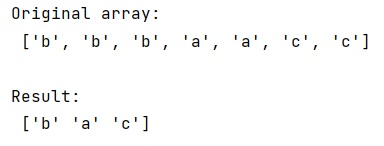# numpy.unique() method with order preserved

Learn about Python's numpy.unique() method with order preserved, how to preserve the order of the result returned by numpy.unique() method?
Submitted by Pranit Sharma, on January 25, 2023

NumPy is an abbreviated form of Numerical Python. It is used for different types of scientific operations in python. Numpy is a vast library in python which is used for almost every kind of scientific or mathematical operation. It is itself an array which is a collection of various methods and functions for processing the arrays.

## numpy.unique() Method

The numpy.unique() method is used to find the unique values of the array i.e. it returns an array that does not contain the repeated set of values.

## numpy.unique() method with order preserved

To preserve the order of the values after applying the unique() method of the array, we should use the return_index property of numpy.unique(). It returns the indices at which the elements first occurred in the input. Then we will use argsort to sort those indices so that the original order will be retained.

Let us understand with the help of an example,

## Python program to preserve order of the result returned by numpy.unique() method

```# Import numpy
import numpy as np

# Creating a numpy array
arr = ['b','b','b','a','a','c','c']

# Display original array
print("Original array:\n",arr,"\n")

# Applying unique function on array
res,ind = np.unique(arr, return_index=True)

# Sorting indices
result = res[np.argsort(ind)]

# Display result
print("Result:\n",result)
```

## Output

The output of the above program is: